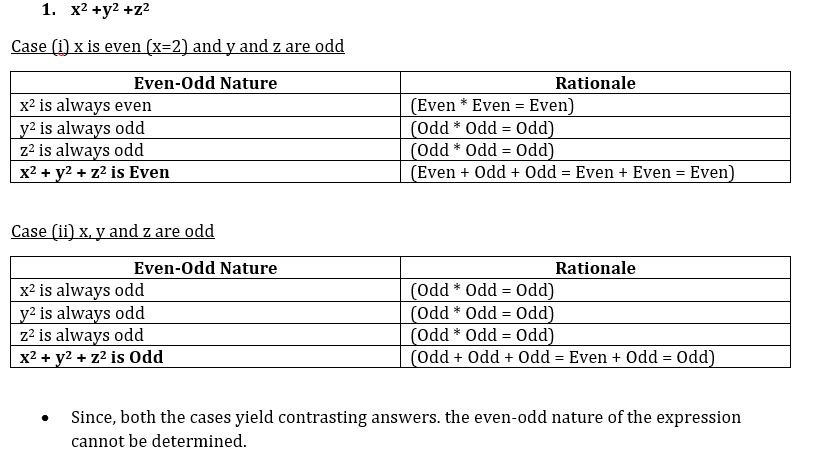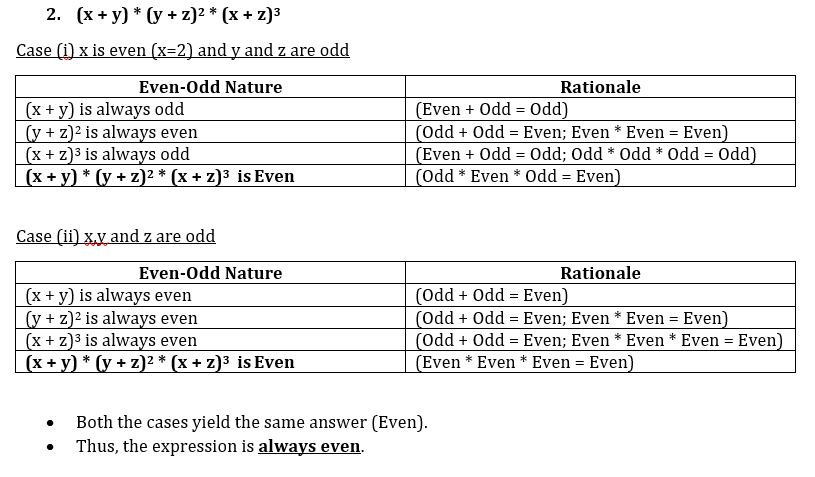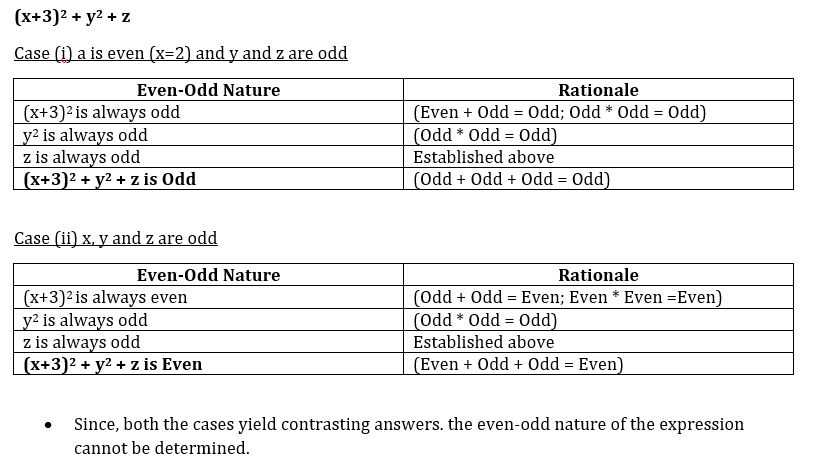GMAT Question of the Day - Daily to your Mailbox; hard ones only

 It is currently 17 Jun 2019, 00:34GMAT Club Daily Prep

Thank you for using the timer - this advanced tool can estimate your performance and suggest more practice questions. We have subscribed you to Daily Prep Questions via email.

Customized
for You

we will pick new questions that match your level based on your Timer History

Track

every week, we’ll send you an estimated GMAT score based on your performance

Practice
Pays

we will pick new questions that match your level based on your Timer History

Not interested in getting valuable practice questions and articles delivered to your email? No problem, unsubscribe here.If x, y, z are three consecutive prime numbers

Author Message
TAGS:

Hide Tags

e-GMAT RepresentativeD
Joined: 04 Jan 2015
Posts: 2888
If x, y, z are three consecutive prime numbers  [#permalink]

Show Tags00:00

Difficulty:55% (hard)

Question Stats:62% (02:08) correct38% (01:57) wrongbased on 178 sessions

HideShow timer Statistics

e-GMAT Question:

If x, y, z are three consecutive prime numbers where x<y<z, which of the following is even?

i) $$x^2+y^2+z^2$$
ii) $$(x + y) * (y + z)^2 * (x + z)^3$$
iii) $$(x+3)^2 + y^2 + z$$

A) Only I
B) Only II
C) Only III
D) Both I and II
E) Both II and III

This is

Question 3 of The e-GMAT Number Properties Marathon

Go to

Question 4 of the Marathon

_________________

Originally posted by EgmatQuantExpert on 27 Feb 2018, 09:36.
Last edited by EgmatQuantExpert on 13 Aug 2018, 02:37, edited 2 times in total.
InternB
Joined: 16 Jan 2018
Posts: 11
Concentration: Strategy, General Management
GMAT 1: 630 Q48 V28GPA: 3.5
Re: If x, y, z are three consecutive prime numbers  [#permalink]

Show Tags

B) Only II, is the correct answer.

i) try to put x=2, y=3 and z=5 => E
Now try to put x=3, y=5 and z=7 => O

ii) this expression will always have one summation even so the complete expression will be even for all the values of x, y and z.

iii) repeat the process done for i, once it will be Odd and in the other it will be even.

Sent from my [device_name] using [url]GMAT Club Forum mobile app[/url]
CEOV
Joined: 12 Sep 2015
Posts: 3777
Re: If x, y, z are three consecutive prime numbers  [#permalink]

Show Tags

Top Contributor
EgmatQuantExpert wrote:

Question:

If x, y, z are three consecutive prime numbers where x<y<z, which of the following is even?

i) x² + y² +z²
ii) (x + y)(y + z)²(x + z)³
iii) (x + 3)² + y² + z

A) Only I
B) Only II
C) Only III
D) Both I and II
E) Both II and III

Test some sets of values
Let's start with x = 2, y = 3 and z = 5
We get:
i) x² + y² + z² = 2² + 3² + 5² = 4 + 9 + 25 = 38 = EVEN
ii) (x + y)(y + z)²(x + z)³ = (2 + 3)(3 + 5)²(2 + 5)³ = (5)(8)²(7)³ = EVEN
iii) (x + 3)² + y² + z = (2 + 3)² + 3² + 5 = 25 + 9 + 5 = 39 ODD
Since iii) is not true, we can ELIMINATE C and E

Now let's test x = 3, y = 5 and z = 7
We get:
i) x² + y² + z² = 3² + 5² + 7² = 9 + 25 + 49 = 83 = ODD
Since i) is not true, we can ELIMINATE A and D

By the process of elimination, we are left with B

RELATED VIDEO FROM OUR COURSE

_________________
e-GMAT RepresentativeD
Joined: 04 Jan 2015
Posts: 2888
Re: If x, y, z are three consecutive prime numbers  [#permalink]

Show Tags

Solution:

• As per the question stem, x, y, and z are three consecutive prime numbers.
• Since prime numbers can be even or odd, there can be two possibilities, depending on the values that x, y, and z can take:
1. One of the prime numbers is even and other two are odd
a. In this case, x has to be 2, since it is the smallest prime number among x, y and z.
b. y and z would be 3 and 5 respectively.
2. All the three prime numbers are odd.
a. This means that all the prime numbers are greater than or equal to 3.
b. For example: x = 5, y = 7, z = 11

Keeping these 2 possibilities in mind, let us analyze each of the 3 expressions:Only expression II is even in nature, and hence the correct answer is Option B.
_________________
Math ExpertV
Joined: 02 Aug 2009
Posts: 7743
Re: If x, y, z are three consecutive prime numbers  [#permalink]

Show Tags

EgmatQuantExpert wrote:

Question:

If x, y, z are three consecutive prime numbers where x<y<z, which of the following is even?

i) $$x^2+y^2+z^2$$
ii) $$(x + y) * (y + z)^2 * (x + z)^3$$
iii) $$(x+3)^2 + y^2 + z$$

A) Only I
B) Only II
C) Only III
D) Both I and II
E) Both II and III

firstly what all can be the cases for x<y<z
1) x is 2 so even and y and z will be odd
2) all three are odd

so y and z are ODD for sure

lets check the equations..

i) $$x^2+y^2+z^2$$
a) if x is even and other two odd, E+O+O=E
b) if all three odd, O+O+O=O

so need not be true
..
ii) $$(x + y) * (y + z)^2 * (x + z)^3$$
a) if x is even and other two odd, y+z will be even..so O*E*O=E
b) if all three odd, E*E*E=E..

Always true
..
iii) $$(x+3)^2 + y^2 + z$$
a) if x is even and other two odd, O+O+O=E
b) if all three odd, E+O+O=O

need not be true

only ii

B
_________________
Senior ManagerS
Joined: 12 Sep 2017
Posts: 263
Re: If x, y, z are three consecutive prime numbers  [#permalink]

Show Tags

Hello!

Shouldn't be written "must be" in order not to consider I?Re: If x, y, z are three consecutive prime numbers   [#permalink] 19 Feb 2019, 12:05
Display posts from previous: Sort by

If x, y, z are three consecutive prime numbers